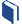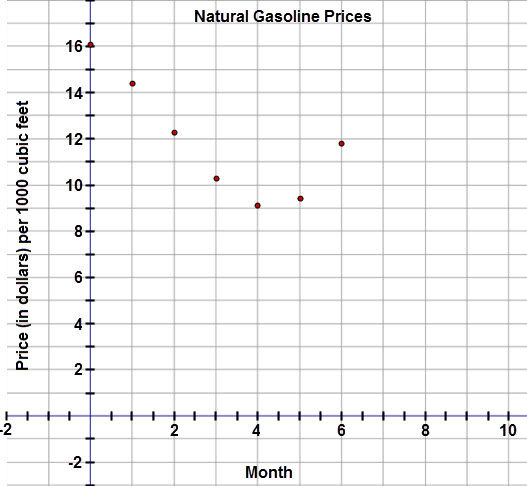# Exploring Other Function Models

###Resources for this lesson:

You will use your Algebra II Journalon this page.

> Glossary> Calculator Resources> Teacher Resources: Instructional NotesWhy might a quadratic regression equation be a good fit for the data set?

Unlike linear and exponential functions, the quadratic function allows for a change in direction.In this data, the price of the natural gas shows a decrease, but after 4 months the price begins to rise.  This indicates that a quadratic model would be a better fit than a linear or exponential model.